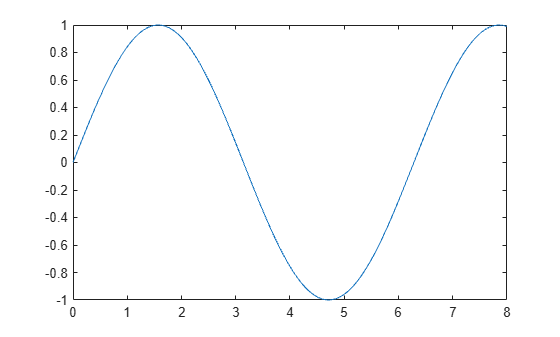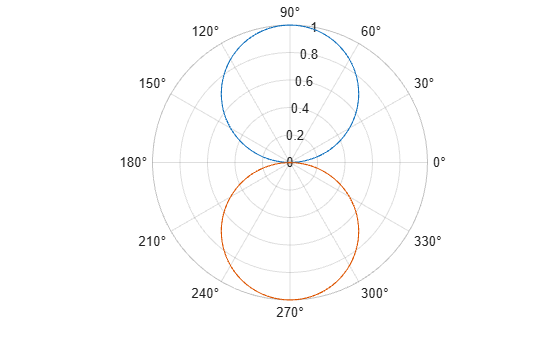# refreshdata

## 语法

``refreshdata``
``refreshdata(target)``
``refreshdata(target,workspace)``

## 说明

``refreshdata` 更新当前图窗中的图数据以反映工作区变量的变化。在使用 `refreshdata` 之前，您必须首先设置图的数据源属性（例如，`XDataSource`）。该函数会在更新图之前计算这些数据源。`

``refreshdata(target)` 使用数据源属性更新指定的图窗、坐标区或图中的图数据。`
``refreshdata(target,workspace)` 指定计算数据源属性的工作区。`

## 示例

```x = linspace(0,8); y = sin(x); ln = plot(x,y);``````ln.XDataSource = 'x'; ln.YDataSource = 'y'; y = sin(3.*x); refreshdata``````theta = linspace(0,2*pi); rho1 = sin(theta); rho2 = -sin(theta); px = polaraxes; hold on; ln1 = polarplot(theta,rho1); ln2 = polarplot(theta,rho2);``````ln1.RDataSource = 'rho1'; ln2.RDataSource = 'rho2'; rho1 = 0.5*sin(theta); rho2 = 0.5*cos(theta);```

`refreshdata(ln1)`## 输入参数

• `'base'` - 在基础工作区中计算数据源属性。

• `'caller'` - 在调用 `refreshdata` 的函数的工作区中计算数据源属性。